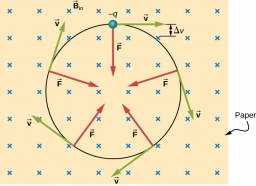# Followed 43871

At 6.40, a cyclist started from the station at a speed of 12 km / h. At exactly 10:00, the car followed him at a speed of 84 km / h. What time does the car catch the cyclist?

Result

t = 10:33 hh:mm### Step-by-step explanation:Did you find an error or inaccuracy? Feel free to write us. Thank you!

Tips for related online calculators
Do you have a linear equation or system of equations and looking for its solution? Or do you have a quadratic equation?
Do you want to convert velocity (speed) units?
Do you want to convert time units like minutes to seconds?